# partialDependence

Compute partial dependence

Since R2020b

## Syntax

``pd = partialDependence(RegressionMdl,Vars)``
``pd = partialDependence(ClassificationMdl,Vars,Labels)``
``pd = partialDependence(___,Data)``
``pd = partialDependence(fun,Vars,Data)``
``pd = partialDependence(___,Name,Value)``
``[pd,x,y] = partialDependence(___)``

## Description

example

````pd = partialDependence(RegressionMdl,Vars)` computes the partial dependence `pd` between the predictor variables listed in `Vars` and the responses predicted by using the regression model `RegressionMdl`, which contains predictor data.```

example

````pd = partialDependence(ClassificationMdl,Vars,Labels)` computes the partial dependence `pd` between the predictor variables listed in `Vars` and the scores for the classes specified in `Labels` by using the classification model `ClassificationMdl`, which contains predictor data.```

example

````pd = partialDependence(___,Data)` uses new predictor data in `Data`. You can specify `Data` in addition to any of the input argument combinations in the previous syntaxes.```

example

````pd = partialDependence(fun,Vars,Data)` computes the partial dependence between the predictor variables listed in `Vars` and the outputs returned by the custom model `fun`, using the predictor data `Data`.```

example

````pd = partialDependence(___,Name,Value)` uses additional options specified by one or more name-value arguments. For example, if you specify `"UseParallel","true"`, the `partialDependence` function uses parallel computing to perform the partial dependence calculations.```
````[pd,x,y] = partialDependence(___)` also returns `x` and `y`, which contain the query points of the first and second predictor variables in `Vars`, respectively. If you specify one variable in `Vars`, then `partialDependence` returns an empty matrix (`[]`) for `y`.```

## Examples

collapse all

Train a naive Bayes classification model with the `fisheriris` data set, and compute partial dependence values that show the relationship between the predictor variable and the predicted scores (posterior probabilities) for multiple classes.

Load the `fisheriris` data set, which contains species (`species`) and measurements (`meas`) on sepal length, sepal width, petal length, and petal width for 150 iris specimens. The data set contains 50 specimens from each of three species: setosa, versicolor, and virginica.

`load fisheriris`

Train a naive Bayes classification model with `species` as the response and `meas` as predictors.

`Mdl = fitcnb(meas,species,"PredictorNames",["Sepal Length","Sepal Width","Petal Length","Petal Width"]);`

Compute partial dependence values on the third predictor variable (petal length) of the scores predicted by `Mdl` for all three classes of `species`. Specify the class labels by using the `ClassNames` property of `Mdl`.

`[pd,x] = partialDependence(Mdl,3,Mdl.ClassNames);`

pd contains the partial dependence values for the query points x. You can plot the computed partial dependence values by using plotting functions such as `plot` and `bar`. Plot `pd` against `x` by using the `bar` function.

```bar(x,pd) legend(Mdl.ClassNames) xlabel("Petal Length") ylabel("Scores") title("Partial Dependence Plot")```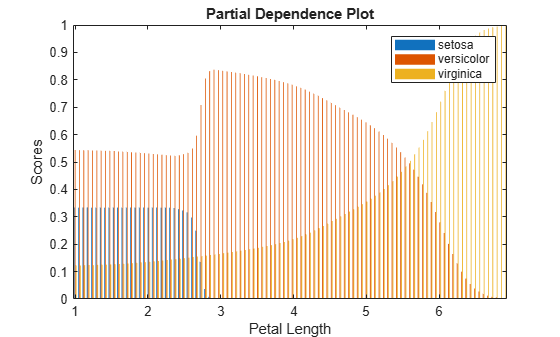According to this model, the probability of `virginica` increases with petal length. The probability of `setosa` is about 0.33, from where petal length is 0 to around 2.5, and then the probability drops to almost 0.

Alternatively, you can use the `plotPartialDependence` function to compute and plot partial dependence values.

`plotPartialDependence(Mdl,3,Mdl.ClassNames)`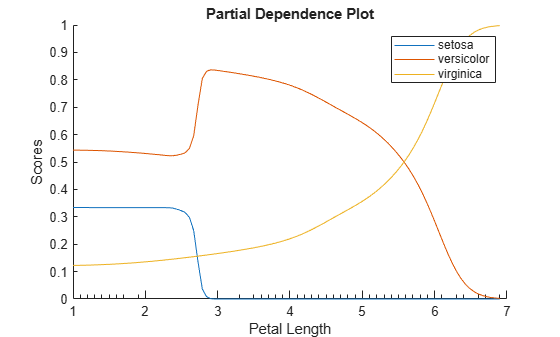Train an ensemble of classification models and compute partial dependence values on two variables for multiple classes. Then plot the partial dependence values for each class.

Load the `census1994` data set, which contains US yearly salary data, categorized as `<=50K` or `>50K`, and several demographic variables.

`load census1994`

Extract a subset of variables to analyze from the table `adultdata`.

```X = adultdata(1:500,["age","workClass","education_num","marital_status","race", ... "sex","capital_gain","capital_loss","hours_per_week","salary"]);```

Train a random forest of classification trees by using `fitcensemble` and specifying `Method` as "`Bag"`. For reproducibility, use a template of trees created by using `templateTree` with the `Reproducible` option.

```rng("default") t = templateTree("Reproducible",true); Mdl = fitcensemble(X,"salary","Method","Bag","Learners",t);```

Inspect the class names in `Mdl`.

`Mdl.ClassNames`
```ans = 2x1 categorical <=50K >50K ```

Compute partial dependence values of the scores on the predictors `age` and `education_num` for both classes (`<=50K` and `>50K`). Specify the number of observations to sample as 100.

`[pd,x,y] = partialDependence(Mdl,["age","education_num"],Mdl.ClassNames,"NumObservationsToSample",100);`

Create a surface plot of the partial dependence values for the first class (`<=50K`) by using the `surf` function.

```figure surf(x,y,squeeze(pd(1,:,:))) xlabel("age") ylabel("education\_num") zlabel("Score of class <=50K") title("Partial Dependence Plot") view([130 30]) % Modify the viewing angle```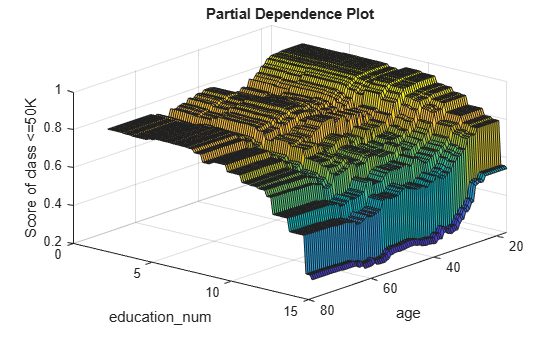Create a surface plot of the partial dependence values for the second class (`>50K`).

```figure surf(x,y,squeeze(pd(2,:,:))) xlabel("age") ylabel("education\_num") zlabel("Score of class >50K") title("Partial Dependence Plot") view([130 30]) % Modify the viewing angle```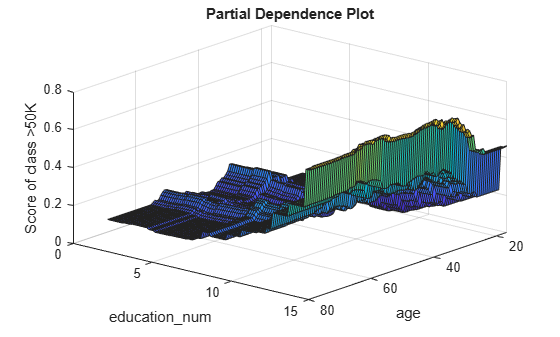The two plots show different partial dependence patterns depending on the class.

Load the `carbig` sample data set.

`load carbig`

The vectors `Displacement`, `Cylinders`, and `Model_Year` contain data for car engine displacement, number of engine cylinders, and year the car was manufactured, respectively.

Fit a multinomial regression model using `Displacement` and `Cylinders` as predictor variables and `Model_Year` as the response.

```predvars = [Displacement,Cylinders]; Mdl = fitmnr(predvars,Model_Year,PredictorNames=["Displacement","Cylinders"]);```

Create a vector of noisy predictor data from the predictor variables by using the `rand` function.

```Data = predvars(1:10:end,:); rng("default") rows = length(Data); Data = Data + 10*rand([rows,2]);```

Calculate the partial dependence of the response category probability corresponding to cars manufactured in 1980 on `Displacement`. Use the noisy predictor data to calculate the partial dependence.

`[pd,x,~] = partialDependence(Mdl,"Displacement",80,Data)`
```pd = 1×100 0.0030 0.0031 0.0031 0.0032 0.0032 0.0033 0.0033 0.0033 0.0034 0.0034 0.0035 0.0035 0.0036 0.0036 0.0036 0.0037 0.0037 0.0037 0.0038 0.0038 0.0038 0.0039 0.0039 0.0039 0.0039 0.0039 0.0040 0.0040 0.0040 0.0040 0.0040 0.0040 0.0040 0.0040 0.0040 0.0040 0.0040 0.0040 0.0040 0.0040 0.0039 0.0039 0.0039 0.0039 0.0038 0.0038 0.0038 0.0037 0.0037 0.0036 ```
```x = 100×1 73.7850 77.1781 80.5713 83.9644 87.3575 90.7507 94.1438 97.5370 100.9301 104.3232 ⋮ ```

The output shows the calculated values for the partial dependence of the category probability on `Displacement`. Because `Displacement` is a continuous variable, the `partialDependence` function calculates the partial dependence at 100 equally spaced query points `x`.

Plot the partial dependence using `plotPartialDependence`.

`plotPartialDependence(Mdl,"Displacement",80,Data)`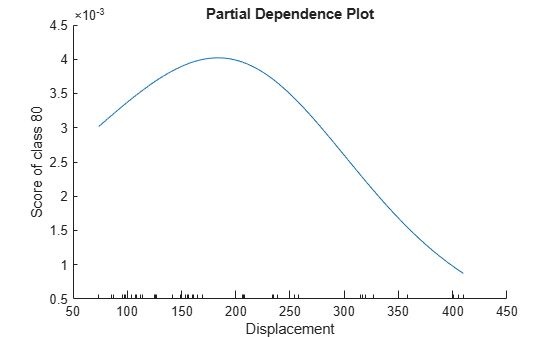The plot shows that when `Displacement` increases from approximately 70 to approximately 180, the probability of a car being manufactured in 1980 increases. As `Displacement` continues to increase, the probability of a car being manufactured in 1980 decreases.

Train a support vector machine (SVM) regression model using the `carsmall` data set, and compute the partial dependence on two predictor variables. Then, create a figure that shows the partial dependence on the two variables along with the histogram on each variable.

Load the `carsmall` data set.

`load carsmall`

Create a table that contains `Weight`, `Cylinders`, `Displacement`, and `Horsepower`.

`Tbl = table(Weight,Cylinders,Displacement,Horsepower);`

Train an SVM regression model using the predictor variables in `Tbl` and the response variable `MPG`. Use a Gaussian kernel function with an automatic kernel scale.

```Mdl = fitrsvm(Tbl,MPG,"ResponseName","MPG", ... "CategoricalPredictors","Cylinders","Standardize",true, ... "KernelFunction","gaussian","KernelScale","auto");```

Compute the partial dependence of the predicted response (`MPG`) on the predictor variables `Weight` and `Horsepower`. Specify query points to compute the partial dependence by using the `QueryPoints` name-value argument.

```numPoints = 10; ptX = linspace(min(Weight),max(Weight),numPoints)'; ptY = linspace(min(Horsepower),max(Horsepower),numPoints)'; [pd,x,y] = partialDependence(Mdl,["Weight","Horsepower"],"QueryPoints",[ptX ptY]);```

Create a figure that contains a 5-by-5 tiled chart layout. Plot the partial dependence on the two variables by using the `imagesc` function. Then draw the histogram for each variable by using the `histogram` function. Specify the edges of the histograms so that the centers of the histogram bars align with the query points. Change the axes properties to align the axes of the plots.

```t = tiledlayout(5,5,"TileSpacing","compact"); ax1 = nexttile(2,[4,4]); imagesc(x,y,pd) title("Partial Dependence Plot") colorbar("eastoutside") ax1.YDir = "normal"; ax2 = nexttile(22,[1,4]); dX = diff(ptX(1:2)); edgeX = [ptX-dX/2;ptX(end)+dX]; histogram(Weight,edgeX); xlabel("Weight") xlim(ax1.XLim); ax3 = nexttile(1,[4,1]); dY = diff(ptY(1:2)); edgeY = [ptY-dY/2;ptY(end)+dY]; histogram(Horsepower,edgeY) xlabel("Horsepower") xlim(ax1.YLim); ax3.XDir = "reverse"; camroll(-90)```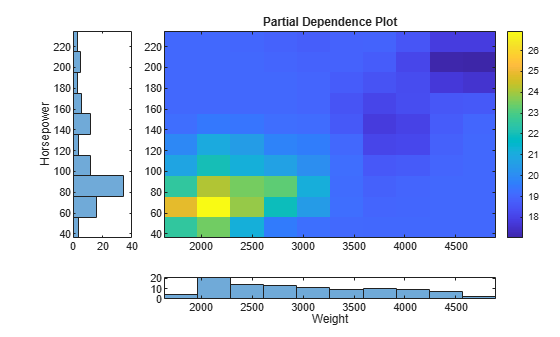Each element of `pd` specifies the color for one pixel of the image plot. The histograms aligned with the axes of the image show the distribution of the predictors.

Compute the partial dependence of label scores on predictor variables for a `SemiSupervisedSelfTrainingModel` object. You cannot pass a `SemiSupervisedSelfTrainingModel` object directly to the `partialDependence` function. Instead, define a custom function that returns label scores for the object, and then pass the function to `partialDependence`.

Randomly generate 15 observations of labeled data, with five observations in each of three classes.

```rng("default") % For reproducibility labeledX = [randn(5,2)*0.25 + ones(5,2); randn(5,2)*0.25 - ones(5,2); randn(5,2)*0.5]; Y = [ones(5,1); ones(5,1)*2; ones(5,1)*3];```

Randomly generate 300 additional observations of unlabeled data, with 100 observations per class.

```unlabeledX = [randn(100,2)*0.25 + ones(100,2); randn(100,2)*0.25 - ones(100,2); randn(100,2)*0.5];```

Fit labels to the unlabeled data by using a semi-supervised self-training method. The function `fitsemiself` returns a `SemiSupervisedSelfTrainingModel` object.

`Mdl = fitsemiself(labeledX,Y,unlabeledX);`

Define the custom function `myLabelScores`, which returns label scores computed by the `predict` function of `SemiSupervisedSelfTrainingModel`; the custom function definition appears at the end of this example.

Compute the partial dependence of the scores for `unlabeledX` on each variable for all classes. `partialDependence` accepts a custom model in the form of a function handle. The function represented by the function handle must accept predictor data and return a column vector or matrix with one row for each observation. Specify the custom model as `@(X)myLabelScores(Mdl,X)` so that the custom function uses the trained model `Mdl` and accepts predictor data.

```[pd1,x1] = partialDependence(@(X)myLabelScores(Mdl,X),1,unlabeledX); [pd2,x2] = partialDependence(@(X)myLabelScores(Mdl,X),2,unlabeledX);```

You can plot the computed partial dependence values by using plotting functions such as `plot` and `bar`. Alternatively, you can use the `plotPartialDependence` function to compute and plot partial dependence values.

Create partial dependence plots for the first variable and all classes.

```plotPartialDependence(@(X)myLabelScores(Mdl,X),1,unlabeledX) xlabel("1st Variable of unlabeledX") ylabel("Scores") legend("Class 1","Class 2","Class 3")```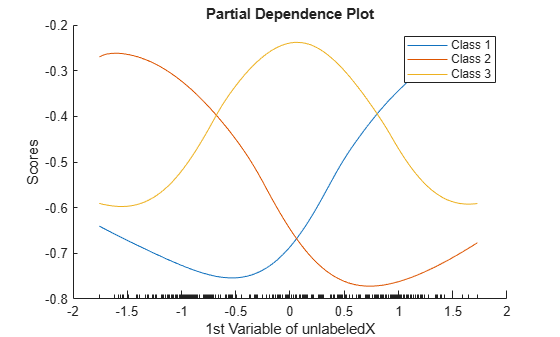Custom Function `myLabelScores`

```function scores = myLabelScores(Mdl,X) [~,scores] = predict(Mdl,X); end```

## Input Arguments

collapse all

Regression model, specified as a full or compact regression model object, as given in the following tables of supported models.

If `RegressionMdl` is a model object that does not contain predictor data (for example, a compact model), you must provide the input argument `Data`.

`partialDependence` does not support a model object trained with a sparse matrix. When you train a model, use a full numeric matrix or table for predictor data where rows correspond to individual observations.

Classification model, specified as a full or compact classification model object, as given in the following table of supported models.

ModelFull or Compact Model Object
Discriminant analysis classifier`ClassificationDiscriminant`, `CompactClassificationDiscriminant`
Multiclass model for support vector machines or other classifiers`ClassificationECOC`, `CompactClassificationECOC`
Ensemble of learners for classification`ClassificationEnsemble`, `CompactClassificationEnsemble`, `ClassificationBaggedEnsemble`
Generalized additive model (GAM)`ClassificationGAM`, `CompactClassificationGAM`
Gaussian kernel classification model using random feature expansion`ClassificationKernel`
k-nearest neighbor classifier`ClassificationKNN`
Linear classification model`ClassificationLinear`
Multiclass naive Bayes model`ClassificationNaiveBayes`, `CompactClassificationNaiveBayes`
Neural network classifier`ClassificationNeuralNetwork`, `CompactClassificationNeuralNetwork`
Support vector machine (SVM) classifier for one-class and binary classification`ClassificationSVM`, `CompactClassificationSVM`
Binary decision tree for multiclass classification`ClassificationTree`, `CompactClassificationTree`
Bagged ensemble of decision trees`TreeBagger`, `CompactTreeBagger`
Multinomial regression model`MultinomialRegression`

If `ClassificationMdl` is a model object that does not contain predictor data (for example, a compact model), you must provide the input argument `Data`.

`partialDependence` does not support a model object trained with a sparse matrix. When you train a model, use a full numeric matrix or table for predictor data where rows correspond to individual observations.

Custom model, specified as a function handle. The function handle `fun` must represent a function that accepts the predictor data `Data` and returns an output in the form of a column vector or matrix. Each row of the output must correspond to each observation (row) in the predictor data.

By default, `partialDependence` uses all output columns of `fun` for the partial dependence computation. You can specify which output columns to use by setting the `OutputColumns` name-value argument.

If the predictor data (`Data`) is in a table, `partialDependence` assumes that a variable is categorical if it is a logical vector, categorical vector, character array, string array, or cell array of character vectors. If the predictor data is a matrix, `partialDependence` assumes that all predictors are continuous. To identify any other predictors as categorical predictors, specify them by using the `CategoricalPredictors` name-value argument.

Data Types: `function_handle`

Predictor variables, specified as a vector of positive integers, character vector, string scalar, string array, or cell array of character vectors. You can specify one or two predictor variables, as shown in the following tables.

One Predictor Variable

ValueDescription
positive integerIndex value corresponding to the column of the predictor data.
character vector or string scalar

Name of the predictor variable. The name must match the entry in the `PredictorNames` property for `RegressionMdl` and `ClassificationMdl` or the variable name of `Data` in a table for a custom model `fun`.

Two Predictor Variables

ValueDescription
vector of two positive integersIndex values corresponding to the columns of the predictor data.
string array or cell array of character vectors

Names of the predictor variables. Each element in the array is the name of a predictor variable. The names must match the entries in the `PredictorNames` property for `RegressionMdl` and `ClassificationMdl` or the variable names of `Data` in a table for a custom model `fun`.

Example: `["x1","x3"]`

Data Types: `single` | `double` | `char` | `string` | `cell`

Class labels, specified as a categorical or character array, logical or numeric vector, or cell array of character vectors. The values and data types in `Labels` must match those of the class names in the `ClassNames` property of `ClassificationMdl` (`ClassificationMdl.ClassNames`).

You can specify one or multiple class labels.

This argument is valid only when you specify a classification model object `ClassificationMdl`.

Example: `["red","blue"]`

Example: `ClassificationMdl.ClassNames([1 3])` specifies `Labels` as the first and third classes in `ClassificationMdl`.

Data Types: `single` | `double` | `logical` | `char` | `cell` | `categorical`

Predictor data, specified as a numeric matrix or table. Each row of `Data` corresponds to one observation, and each column corresponds to one variable.

For both a regression model (`RegressionMdl`) and a classification model (`ClassificationMdl`), `Data` must be consistent with the predictor data that trained the model, stored in either the `X` or `Variables` property.

• If you trained the model using a numeric matrix, then `Data` must be a numeric matrix. The variables that make up the columns of `Data` must have the same number and order as the predictor variables that trained the model.

• If you trained the model using a table (for example, `Tbl`), then `Data` must be a table. All predictor variables in `Data` must have the same variable names and data types as the names and types in `Tbl`. However, the column order of `Data` does not need to correspond to the column order of `Tbl`.

• `Data` must not be sparse.

If you specify a regression or classification model that does not contain predictor data, you must provide `Data`. If the model is a full model object that contains predictor data and you specify the `Data` argument, then `partialDependence` ignores the predictor data in the model and uses `Data` only.

If you specify a custom model `fun`, you must provide `Data`.

Data Types: `single` | `double` | `table`

### Name-Value Arguments

Specify optional pairs of arguments as `Name1=Value1,...,NameN=ValueN`, where `Name` is the argument name and `Value` is the corresponding value. Name-value arguments must appear after other arguments, but the order of the pairs does not matter.

Before R2021a, use commas to separate each name and value, and enclose `Name` in quotes.

Example: `partialDependence(Mdl,Vars,Data,"NumObservationsToSample",100,"UseParallel",true)` computes the partial dependence values by using 100 sampled observations in `Data` and executing `for`-loop iterations in parallel.

Flag to include interaction terms of the generalized additive model (GAM) in the partial dependence computation, specified as `true` or `false`. This argument is valid only for a GAM. That is, you can specify this argument only when `RegressionMdl` is `RegressionGAM` or `CompactRegressionGAM`, or `ClassificationMdl` is `ClassificationGAM` or `CompactClassificationGAM`.

The default `IncludeInteractions` value is `true` if the model contains interaction terms. The value must be `false` if the model does not contain interaction terms.

Example: `"IncludeInteractions",false`

Data Types: `logical`

Flag to include an intercept term of the generalized additive model (GAM) in the partial dependence computation, specified as `true` or `false`. This argument is valid only for a GAM. That is, you can specify this argument only when `RegressionMdl` is `RegressionGAM` or `CompactRegressionGAM`, or `ClassificationMdl` is `ClassificationGAM` or `CompactClassificationGAM`.

Example: `"IncludeIntercept",false`

Data Types: `logical`

Number of observations to sample, specified as a positive integer. The default value is the number of total observations in `Data` or the model (`RegressionMdl` or `ClassificationMdl`). If you specify a value larger than the number of total observations, then `partialDependence` uses all observations.

`partialDependence` samples observations without replacement by using the `datasample` function and uses the sampled observations to compute partial dependence.

Example: `"NumObservationsToSample",100`

Data Types: `single` | `double`

Points to compute partial dependence for numeric predictors, specified as a numeric column vector, a numeric two-column matrix, or a cell array of two numeric column vectors.

• If you select one predictor variable in `Vars`, use a numeric column vector.

• If you select two predictor variables in `Vars`:

• Use a numeric two-column matrix to specify the same number of points for each predictor variable.

• Use a cell array of two numeric column vectors to specify a different number of points for each predictor variable.

The default value is a numeric column vector or a numeric two-column matrix, depending on the number of selected predictor variables. Each column contains 100 evenly spaced points between the minimum and maximum values of the sampled observations for the corresponding predictor variable.

You cannot modify `QueryPoints` for a categorical variable. The `partialDependence` function uses all categorical values in the selected variable.

If you select one numeric variable and one categorical variable, you can specify `QueryPoints` for a numeric variable by using a cell array consisting of a numeric column vector and an empty array.

Example: `"QueryPoints",{pt,[]}`

Data Types: `single` | `double` | `cell`

Flag to run in parallel, specified as `true` or `false`. If you specify `"UseParallel",true`, the `partialDependence` function executes `for`-loop iterations by using `parfor` when predicting responses or scores for each observation and averaging them. The loop runs in parallel when you have Parallel Computing Toolbox™.

Example: `"UseParallel",true`

Data Types: `logical`

Categorical predictors list for the custom model `fun`, specified as one of the values in this table.

ValueDescription
Vector of positive integers

Each entry in the vector is an index value indicating that the corresponding predictor is categorical. The index values are between 1 and `p`, where `p` is the number of variables in `Data`.

Logical vector

A `true` entry means that the corresponding predictor is categorical. The length of the vector is `p`.

Character matrixEach row of the matrix is the name of a predictor variable. The names must match the variable names of the predictor data `Data` in a table. Pad the names with extra blanks so each row of the character matrix has the same length.
String array or cell array of character vectorsEach element in the array is the name of a predictor variable. The names must match the variable names of the predictor data `Data` in a table.
`"all"`All predictors are categorical.

By default, if the predictor data `Data` is in a table, `partialDependence` assumes that a variable is categorical if it is a logical vector, categorical vector, character array, string array, or cell array of character vectors. If the predictor data is a matrix, `partialDependence` assumes that all predictors are continuous. To identify any other predictors as categorical predictors, specify them by using the `CategoricalPredictors` name-value argument.

This argument is valid only when you specify a custom model by using `fun`.

Example: `"CategoricalPredictors","all"`

Data Types: `single` | `double` | `logical` | `char` | `string` | `cell`

Output columns of the custom model `fun` to use for the partial dependence computation, specified as one of the values in this table.

ValueDescription
Vector of positive integers

Each entry in the vector is an index value indicating that `partialDependence` uses the corresponding output column for the partial dependence computation. The index values are between 1 and `q`, where `q` is the number of columns in the output matrix returned by the custom model `fun`.

Logical vector

A `true` entry means that `partialDependence` uses the corresponding output column for the partial dependence computation. The length of the vector is `q`.

`"all"``partialDependence` uses all output columns for the partial dependence computation.

This argument is valid only when you specify a custom model by using `fun`.

Example: `"OutputColumns",[1 2]`

Data Types: `single` | `double` | `logical` | `char` | `string`

## Output Arguments

collapse all

Partial dependence values, returned as a numeric array.

The dimension of `pd` depends on the type of model (regression, classification or custom), number of variables specified in `Vars`, number of classes specified in `Labels` (classification model only), and number of columns specified in `OutputColumns` (custom model only).

For a regression model (`RegressionMdl`), the following conditions apply:

• If you specify two variables in `Vars`, `pd` is a `numY`-by-`numX` matrix, where `numY` and `numX` are the number of query points of the second and first variables in `Vars`, respectively. The value in `pd(i,j)` is the partial dependence value of the query point corresponding to `y(i)` and `x(j)`. `y(i)` is the `i`th query point of the second predictor variable, and `x(j)` is the `j`th query point of the first predictor variable.

• If you specify one variable in `Vars`, `pd` is a `1`-by-`numX` vector.

For a classification model (`ClassificationMdl`), the following conditions apply:

• If you specify two variables in `Vars`, `pd` is a `num`-by-`numY`-by-`numX` array, where `num` is the number of class labels in `Labels`. The value in `pd(i,j,k)` is the partial dependence value of the query point `y(j)` and `x(k)` for the `i`th class label in `Labels`.

• If you specify one variable in `Vars`, `pd` is a `num`-by-`numX` matrix.

• If you specify one class in `Labels`, `pd` is a `numY`-by-`numX` matrix.

• If you specify one variable and one class, `pd` is a `1`-by-`numX` vector.

For a custom model (`fun`), the following conditions apply:

• If you specify two variables in `Vars`, `pd` is a `num`-by-`numY`-by-`numX` array, where `num` is the number of output columns in `OutputColumns`. The value in `pd(i,j,k)` is the partial dependence value of the query point `y(j)` and `x(k)` for the `i`th column in `OutputColumns`.

• If you specify one variable in `Vars`, `pd` is a `num`-by-`numX` matrix.

• If you specify one column in `OutputColumns`, `pd` is a `numY`-by-`numX` matrix.

• If you specify one variable and one column, `pd` is a `1`-by-`numX` vector.

Query points of the first predictor variable in `Vars`, returned as a numeric or categorical column vector.

If the predictor variable is numeric, then you can specify the query points by using the `QueryPoints` name-value argument.

Data Types: `single` | `double` | `categorical`

Query points of the second predictor variable in `Vars`, returned as a numeric or categorical column vector. This output argument is empty (`[]`) if you specify only one variable in `Vars`.

If the predictor variable is numeric, then you can specify the query points by using the `QueryPoints` name-value argument.

Data Types: `single` | `double` | `categorical`

collapse all

### Partial Dependence for Regression Models

Partial dependence represents the relationships between predictor variables and predicted responses in a trained regression model. `partialDependence` computes the partial dependence of predicted responses on a subset of predictor variables by marginalizing over the other variables.

Consider partial dependence on a subset XS of the whole predictor variable set X = {x1, x2, …, xm}. A subset XS includes either one variable or two variables: XS = {xS1} or XS = {xS1, xS2}. Let XC be the complementary set of XS in X. A predicted response f(X) depends on all variables in X:

f(X) = f(XS, XC).

The partial dependence of predicted responses on XS is defined by the expectation of predicted responses with respect to XC:

`${f}^{S}\left({X}^{S}\right)={E}_{C}\left[f\left({X}^{S},{X}^{C}\right)\right]=\int f\left({X}^{S},{X}^{C}\right){p}_{C}\left({X}^{C}\right)d{X}^{C},$`

where pC(XC) is the marginal probability of XC, that is, ${p}_{C}\left({X}^{C}\right)\approx \int p\left({X}^{S},{X}^{C}\right)d{X}^{S}$. Assuming that each observation is equally likely, and the dependence between XS and XC and the interactions of XS and XC in responses is not strong, `partialDependence` estimates the partial dependence by using observed predictor data as follows:

 ${f}^{S}\left({X}^{S}\right)\approx \frac{1}{N}\sum _{i=1}^{N}f\left({X}^{S},{X}_{i}{}^{C}\right),$ (1)

where N is the number of observations and Xi = (XiS, XiC) is the ith observation.

When you call the `partialDependence` function, you can specify a trained model (f(·)) and select variables (XS) by using the input arguments `RegressionMdl` and `Vars`, respectively. `partialDependence` computes the partial dependence at 100 evenly spaced points of XS or the points that you specify by using the `QueryPoints` name-value argument. You can specify the number (N) of observations to sample from given predictor data by using the `NumObservationsToSample` name-value argument.

### Partial Dependence Classification Models

In the case of classification models, `partialDependence` computes the partial dependence in the same way as for regression models, with one exception: instead of using the predicted responses from the model, the function uses the predicted scores for the classes specified in `Labels`.

### Weighted Traversal Algorithm

The weighted traversal algorithm is a method to estimate partial dependence for a tree-based model. The estimated partial dependence is the weighted average of response or score values corresponding to the leaf nodes visited during the tree traversal.

Let XS be a subset of the whole variable set X and XC be the complementary set of XS in X. For each XS value to compute partial dependence, the algorithm traverses a tree from the root (beginning) node down to leaf (terminal) nodes and finds the weights of leaf nodes. The traversal starts by assigning a weight value of one at the root node. If a node splits by XS, the algorithm traverses to the appropriate child node depending on the XS value. The weight of the child node becomes the same value as its parent node. If a node splits by XC, the algorithm traverses to both child nodes. The weight of each child node becomes a value of its parent node multiplied by the fraction of observations corresponding to each child node. After completing the tree traversal, the algorithm computes the weighted average by using the assigned weights.

For an ensemble of bagged trees, the estimated partial dependence is an average of the weighted averages over the individual trees.

## Algorithms

For both a regression model (`RegressionMdl`) and a classification model (`ClassificationMdl`), `partialDependence` uses a `predict` function to predict responses or scores. `partialDependence` chooses the proper `predict` function according to the model and runs `predict` with its default settings. For details about each `predict` function, see the `predict` functions in the following two tables. If the specified model is a tree-based model (not including a boosted ensemble of trees), then `partialDependence` uses the weighted traversal algorithm instead of the `predict` function. For details, see Weighted Traversal Algorithm.

Regression Model Object

Model TypeFull or Compact Regression Model ObjectFunction to Predict Responses
Bootstrap aggregation for ensemble of decision trees`CompactTreeBagger``predict`
Bootstrap aggregation for ensemble of decision trees`TreeBagger``predict`
Ensemble of regression models`RegressionEnsemble`, `RegressionBaggedEnsemble`, `CompactRegressionEnsemble``predict`
Gaussian kernel regression model using random feature expansion`RegressionKernel``predict`
Gaussian process regression`RegressionGP`, `CompactRegressionGP``predict`
Generalized additive model`RegressionGAM`, `CompactRegressionGAM``predict`
Generalized linear mixed-effect model`GeneralizedLinearMixedModel``predict`
Generalized linear model`GeneralizedLinearModel`, `CompactGeneralizedLinearModel``predict`
Linear mixed-effect model`LinearMixedModel``predict`
Linear regression`LinearModel`, `CompactLinearModel``predict`
Linear regression for high-dimensional data`RegressionLinear``predict`
Neural network regression model`RegressionNeuralNetwork`, `CompactRegressionNeuralNetwork``predict`
Nonlinear regression`NonLinearModel``predict`
Regression tree`RegressionTree`, `CompactRegressionTree``predict`
Support vector machine`RegressionSVM`, `CompactRegressionSVM``predict`

Classification Model Object

Model TypeFull or Compact Classification Model ObjectFunction to Predict Labels and Scores
Discriminant analysis classifier`ClassificationDiscriminant`, `CompactClassificationDiscriminant``predict`
Multiclass model for support vector machines or other classifiers`ClassificationECOC`, `CompactClassificationECOC``predict`
Ensemble of learners for classification`ClassificationEnsemble`, `CompactClassificationEnsemble`, `ClassificationBaggedEnsemble``predict`
Gaussian kernel classification model using random feature expansion`ClassificationKernel``predict`
Generalized additive model`ClassificationGAM`, `CompactClassificationGAM``predict`
k-nearest neighbor model`ClassificationKNN``predict`
Linear classification model`ClassificationLinear``predict`
Naive Bayes model`ClassificationNaiveBayes`, `CompactClassificationNaiveBayes``predict`
Neural network classifier`ClassificationNeuralNetwork`, `CompactClassificationNeuralNetwork``predict`
Support vector machine for one-class and binary classification`ClassificationSVM`, `CompactClassificationSVM``predict`
Binary decision tree for multiclass classification`ClassificationTree`, `CompactClassificationTree``predict`
Bagged ensemble of decision trees`TreeBagger`, `CompactTreeBagger``predict`

## Alternative Functionality

 Friedman, Jerome. H. “Greedy Function Approximation: A Gradient Boosting Machine.” The Annals of Statistics 29, no. 5 (2001): 1189-1232.

 Hastie, Trevor, Robert Tibshirani, and Jerome Friedman. The Elements of Statistical Learning. New York, NY: Springer New York, 2009.